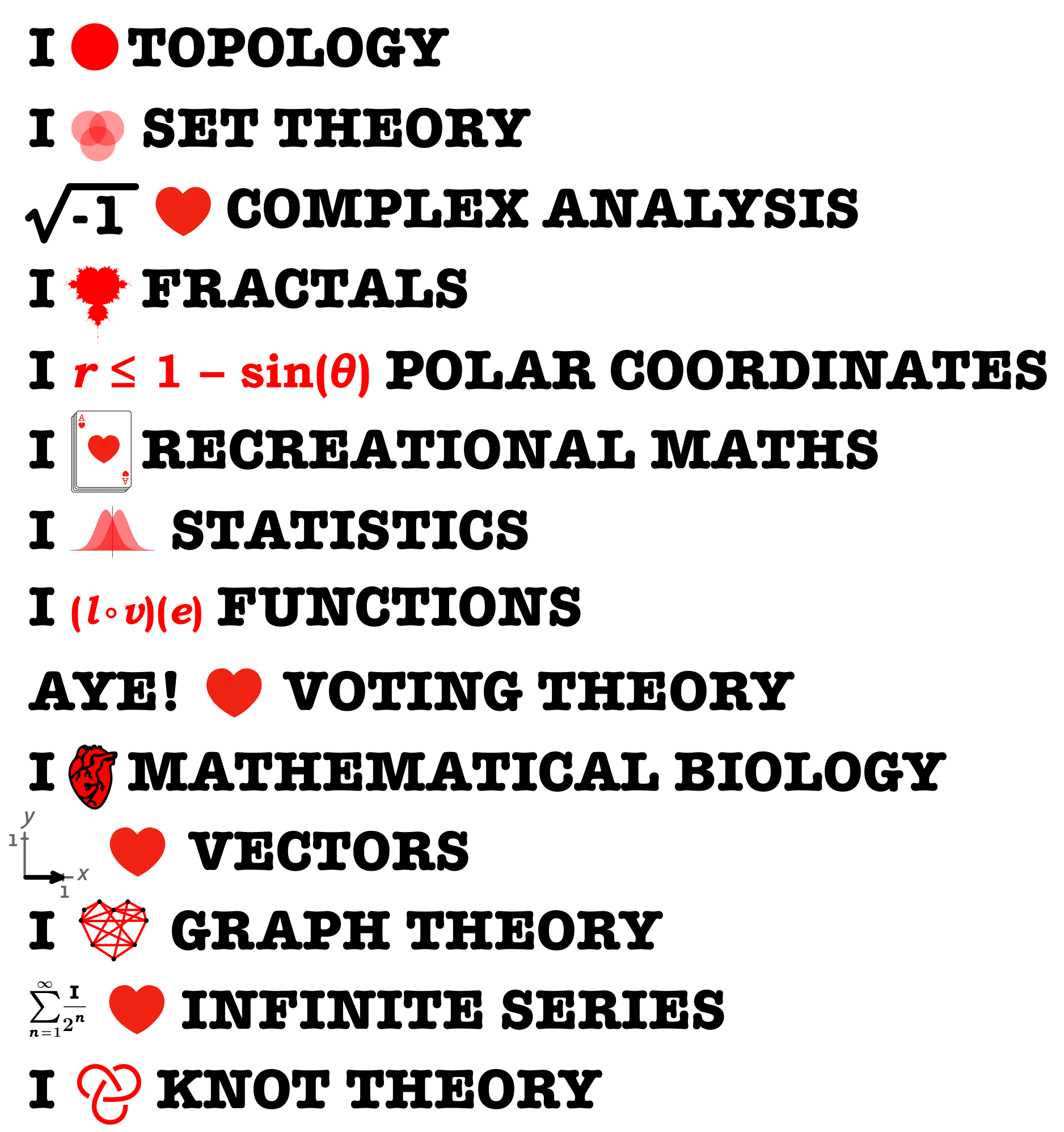# Crossnumber winners, Issue 08

It’s that time of the year again: time to announce the winners of the Chalkdust prize crossnumber #8! Before we reveal the winners, we can reveal the solution and some interesting bits of maths hidden in the clues.
Continue reading# How to make: a Möbius Surprise• A3 paper
• scissors
• glue

#### Instructions

1. Cut your A3 paper into 4 strips.

2. Take two strips and glue them perpendicularly to make a cross.

3. Make a Möbius strip with each of the perpendicular strips.

4. Cut along the centre line of one Möbius strip until you get to where you started. Then cut along the other.

5. Be surprised.# Hilbert’s hotel: the board game# Prize crossnumber, Issue 08

Our original prize crossnumber is featured on pages 54 and 55 of Issue 08.

### Rules

• Although many of the clues have multiple answers, there is only one solution to the completed crossnumber. As usual, no numbers begin with 0. Use of Python, OEIS, Wikipedia, etc. is advised for some of the clues.
• One randomly selected correct answer will win a £100 Maths Gear goody bag, including non-transitive dice, a Festival of the Spoken Word DVD, a dodecaplex puzzle and much, much more. Three randomly selected runners up will win a Chalkdust T-shirt. The prizes have been provided by Maths Gear, a website that sells nerdy things worldwide. Find out more at mathsgear.co.uk
• To enter, submit the sum of the across clues via this form by 2 February 2019. Only one entry per person will be accepted. Winners will be notified by email and announced on our blog by 16 February 2019.# Which number are you?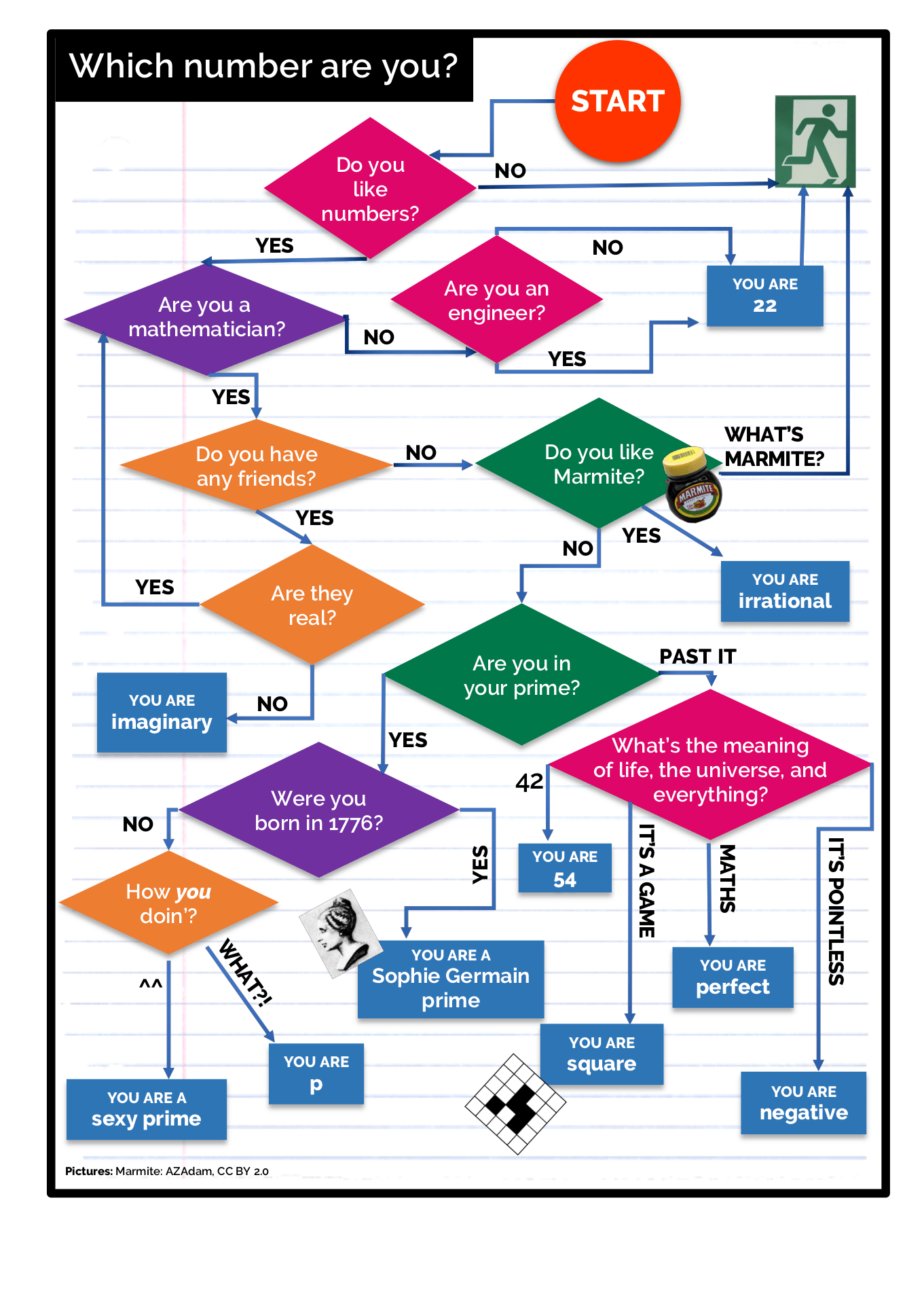# On the cover: Hydrogen orbitals

Quantum mechanics has a reputation.

It’s notorious for being obtuse, difficult, confusing, and unintuitive. That reputation is… entirely deserved. I work on quantum systems full time for my job and I feel like I’ve barely scratched the surface of the mysteries it contains. But one other feature of quantum mechanics that’s often overlooked is how beautiful it can be.

So, for the cover of this issue, I wanted to share one aspect of quantum mechanics that I think is stunning. It’s a certain set of solutions to a differential equation: the orbitals of an electron in a hydrogen atom.

In school, you’re taught that electrons orbit the nucleus of an atom like a planet orbiting a star. This is mostly wrong. The main problem is that electrons, protons and neutrons aren’t little billiard balls, they exist as ‘clouds’ of probability.

To understand what a hydrogen atom really looks like, imagine a cloud of something whizzing around a single proton. The proton’s positive charge attracts and traps the negatively-charged something in what we call the proton’s potential well. Imagine that cloud is denser in some places and sparser in others. That cloud of something can be just one electron whose position has been smeared out. The density of the cloud at a point represents the probability of finding the electron at that point in space. The electron’s position may be smeared out over all space, but it has different odds of being found at different points in space. In fact, it’s usually exponentially less likely to be found outside the small, confined volume of the potential well.

The mathematical explanation for this is that our system is obeying the Schrödinger equation. For our case, it looks like this:

\begin{equation*}
\Big(\dfrac{-\hbar^{\hspace{0.3mm}2}}{2m}\nabla^2 + V(\mathbf{r})\Big)\psi(\mathbf{r}) = E\psi(\mathbf{r}).
\end{equation*}

The Schrödinger equation is the foundational equation of quantum mechanics. It’s used to determine the wavefunction, $\psi(\mathbf{r})$, and the energy, $E$, of the components of the system. In this case the wavefunction represents the electron (with mass $m$) trapped in the electric potential well of the proton, which is represented by $V(\mathbf{r})$. The reduced Planck constant, $\hbar$, (often called “h-bar”) is a fundamental physical constant, and $\nabla^2$ is the Laplacian operator, which sums second derivatives over all the coordinates. The modulus squared of the wavefunction, $\vert\psi(\mathbf{r})\vert^2$, tells you what the density of that probability cloud is like: where are you more likely to find the electron?

Most people who do quantum mechanics for a living spend their time solving this equation and its variants, myself included. The problem is that this is really, really hard. The Schrödinger equation for a hydrogen atom has analytic solutions you can write down, but with almost all other physical systems, you aren’t so lucky. Once you have more than one electron, the complexity skyrockets. Understanding the analytic solutions form an important part of a physics undergraduate’s introduction to quantum mechanics, especially in my field of research. I work on finding approximate solutions to the Schrödinger equation for more complex systems.

To solve the Schrödinger equation, you can separate the wavefunction to get a radial part which is a function of the distance from the nucleus, $r$, and an angular part which is a function of the angles $(\theta, \phi)$. Both parts have multiple solutions, and it turns out that you need three labels to identify these solutions. We call these labels quantum numbers. Here, the three are called $n$, $l$, and $m$. Putting these two concepts together, we can say:

\begin{equation*}
E\psi_{nlm}(\mathbf{r}) = R_n(r)Y_{lm}(\theta, \phi).
\end{equation*}Plots of the solution with $n=0$, $l=5$ and $m=0$ to $m=5$

There’s lots of constraints on the allowed values of $n$, $l$, and $m$, but the most important one is that each number take whole number values only. This is where the ‘quantum’ in quantum mechanics comes from!

The quantum numbers each have physical interpretations: they loosely correspond to the three spatial coordinates. Here, $n$ corresponds to energy. Higher values of $n$ mean the electron has a larger amount of energy, which, due to how electric fields work, also exactly corresponds to a larger distance from the nucleus. That means $n$ is associated with the radial coordinate: the higher $n$ is, the further from the nucleus the electron can be.

Meanwhile, $l$ and $m$ correspond to angular momentum, and so they are associated with the angular coordinates. Roughly speaking, higher values of $l$ correspond to the electron ‘orbiting’ around the nucleus with greater energy (in a weird, quantum mechanical way that doesn’t really look like a planet orbiting a star). Changing $m$ means changing exactly how it orbits for a given value of $l$.

What this all means in practice is that by varying the three quantum numbers you get a huge variety of electron distributions. For instance, $n=1$, $l=0$, $m=0$ means that the electron isn’t orbiting the nucleus at all, so it’s most likely to be found right on top of the nucleus – opposite charges attract! When $n$, $l$, and $m$ are all large you get things like concentric sets of lobes of varying shapes and sizes.

Bringing it back to the cover, the pictures were all generated by making a 2D slice through the full 3D distribution at $y=0$. The brighter a given point is shaded, the higher the value of $\vert\psi(\mathbf{r})\vert^2$ is there—the higher the odds of finding the electron there are. The full 3D versions look like spheres, balloons, lobes, and other wild shapes. The 2D slices have a different sort of haunting beauty to them. The distributions can be concentric rings, orange slices, weird lobes, insect-like segments, and more.

The front cover is the 2D slice of the solution for $n=9$, $l=4$, $m=1$. The back cover contains all the allowed solutions from $n=1$, $l=0$, $m=0$ up to $n=9$, $l=7$, $m=7$.

These orbitals are beautiful by themselves as pieces of abstract maths, but they also provide profound insights into the strange quantum nature of our reality. They’re a testament to the amazing power physics and mathematics can have when they work together to help us understand our universe.# Dear Dirichlet, Issue 08

Moonlighting agony uncle Professor Dirichlet answers your personal problems. Want the prof’s help? Send your problems to deardirichlet@chalkdustmagazine.com.

### Dear Dirichlet,

The annual village fete is fast approaching, and every year I embarrass myself at `guess the number of sweets in the jar’. My exasperated wife ends up telling me to just say a number, and I always panic. Last year my guess was $\mathrm{i} – \text{π}$. Maybe I was just hungry.

— Hungry hungry hippo, Gospel Oak# Top Ten: Units of measurement

This issue, Top Ten features the top ten units of measurement! Then vote here on the top ten Chalkdust regulars for issue 09!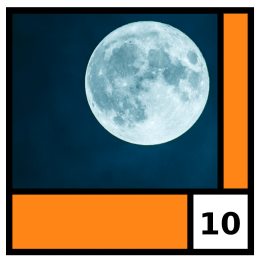At 10, it’s the Zappa.At 9, and selling one tenth of the number of copies that number 6 sold: it’s a millimetre.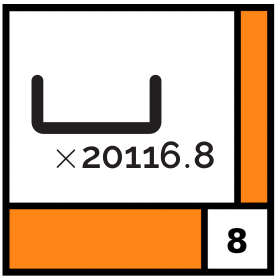At 8, and not receiving much radio play due to being far longer than the rest of the top ten: it’s a furlong.Following warm reviews from critics, degrees Celsius enters the top ten at 7.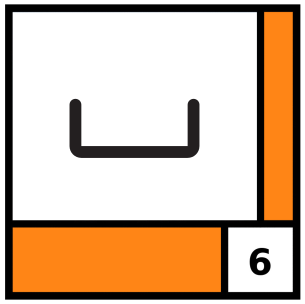The new single by no-one’s favourite rapper 50 Centimetre is at 6.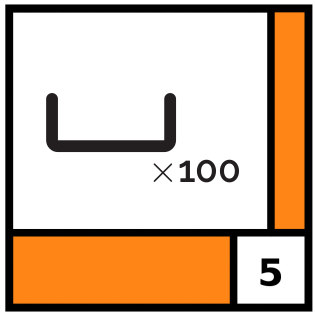At 5, it’s the Yardbirds tribute act whose members are all 8.5cm taller than the originals: the Metrebirds.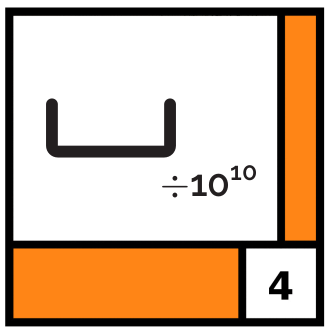Following the release of its 51st anniversary deluxe edition, The Velvet Underground and Picometre is at 4.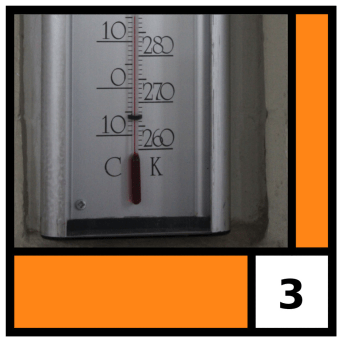At 3, and selling 273.15 more copies than this issue’s number 7: it’s Kelvin Harris.
Image: Wikimedia commons user Martinvl, CC BY-SA 3.0.Forced up two places from last issue, it’s the Newton.Topping the pops this issue, it’s dimensionless constants.# Top ten vote issue 08

What is the best Chalkdust regular?

View ResultsLoading ...# I ❤️ maths

Tired of hearing people ask which area of maths is your favourite? Get one of these tattooed on your forehead and you’ll never be asked again…